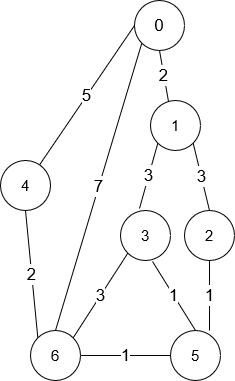Formatted question description: https://leetcode.ca/all/1976.html

# 1976. Number of Ways to Arrive at Destination

Medium

## Description

You are in a city that consists of n intersections numbered from 0 to n - 1 with bi-directional roads between some intersections. The inputs are generated such that you can reach any intersection from any other intersection and that there is at most one road between any two intersections.

You are given an integer n and a 2D integer array roads where roads[i] = [u_i, v_i, time_i] means that there is a road between intersections u_i and v_i that takes time_i minutes to travel. You want to know in how many ways you can travel from intersection 0 to intersection n - 1 in the shortest amount of time.

Return the ** number of ways** you can arrive at your destination in the shortest amount of time. Since the answer may be large, return it modulo 10^9 + 7.

Example 1:Input: n = 7, roads = [[0,6,7],[0,1,2],[1,2,3],[1,3,3],[6,3,3],[3,5,1],[6,5,1],[2,5,1],[0,4,5],[4,6,2]]

Output: 4

Explanation: The shortest amount of time it takes to go from intersection 0 to intersection 6 is 7 minutes.

The four ways to get there in 7 minutes are:

• 0 ➝ 6
• 0 ➝ 4 ➝ 6
• 0 ➝ 1 ➝ 2 ➝ 5 ➝ 6
• 0 ➝ 1 ➝ 3 ➝ 5 ➝ 6

Example 2:

Input: n = 2, roads = [[1,0,10]]

Output: 1

Explanation: There is only one way to go from intersection 0 to intersection 1, and it takes 10 minutes.

Constraints:

• 1 <= n <= 200
• n - 1 <= roads.length <= n * (n - 1) / 2
• roads[i].length == 3
• 0 <= u_i, v_i <= n - 1
• 1 <= time_i <= 10^9
• u_i != v_i
• There is at most one road connecting any two intersections.
• You can reach any intersection from any other intersection.

## Solution

Calculate the shortest time to arrive at each intersection from intersection 0 using Dijkstra’s algorithm. Then starting from intersection 0, calculate the number of ways to arrive at each intersection with the shortest time. Finally, return the number of ways to arrive at intersection n - 1.

class Solution {
public int countPaths(int n, int[][] roads) {
final int MODULO = 1000000007;
Map<Integer, List<int[]>> map = new HashMap<Integer, List<int[]>>();
int u = road, v = road, time = road;
List<int[]> list1 = map.getOrDefault(u, new ArrayList<int[]>());
List<int[]> list2 = map.getOrDefault(v, new ArrayList<int[]>());
map.put(u, list1);
map.put(v, list2);
}
long[] times = new long[n];
Arrays.fill(times, Long.MAX_VALUE);
times = 0;
PriorityQueue<Integer> priorityQueue = new PriorityQueue<Integer>(new Comparator<Integer>() {
public int compare(Integer num1, Integer num2) {
long difference = times[num1] - times[num2];
if (difference > 0)
return 1;
else if (difference < 0)
return -1;
else
return 0;
}
});
priorityQueue.offer(0);
while (!priorityQueue.isEmpty()) {
int curNode = priorityQueue.poll();
long curTime = times[curNode];
List<int[]> list = map.getOrDefault(curNode, new ArrayList<int[]>());
for (int[] road : list) {
int nextNode = road, nextTime = road;
long nextTotalTime = curTime + nextTime;
if (nextTotalTime < times[nextNode]) {
times[nextNode] = nextTotalTime;
priorityQueue.offer(nextNode);
}
}
}
boolean[] visited = new boolean[n];
int[] counts = new int[n];
visited = true;
counts = 1;
priorityQueue.offer(0);
while (!priorityQueue.isEmpty()) {
int size = priorityQueue.size();
int curNode = priorityQueue.poll();
long curTime = times[curNode];
int curCount = counts[curNode];
List<int[]> list = map.getOrDefault(curNode, new ArrayList<int[]>());
for (int[] road : list) {
int nextNode = road, nextTime = road;
long nextTotalTime = curTime + nextTime;
if (nextTotalTime == times[nextNode]) {
counts[nextNode] = (counts[nextNode] + curCount) % MODULO;
if (!visited[nextNode]) {
visited[nextNode] = true;
priorityQueue.offer(nextNode);
}
}
}
}
return counts[n - 1];
}
}